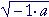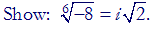S k i l l
i n
A L G E B R A

30

# COMPLEX OR IMAGINARY NUMBERS

The defining property of i

IN ALGEBRA, we want to be able to say that every polynomial equation has a solution; specifically, this one:

x2 + 1 = 0.

That implies,

x2 = −1.

But there is no real number whose square is negative. (Lesson 13.) Therefore, we invent a number -- a complex or imaginary number -- and we call it i.

i2  =  −1.

That is the defining property of the complex unit i.  Its square is negative.

In other words,

i  =.

The complex number i is purely algebraic. That is, we call it a "number" because it will obey all the rules we normally associate with a number. We may add it, subtract it, multiply it, and so on. The complex number i turns out to be extremely useful in mathematics and physics.

Example 1.   3i· 4i = 12i2 = 12(−1) = −12.

Example 2.   −5i· 6i = −30i2 = 30.

We see, then, that the factor i2 changes the sign of a product.

Problem 1.   Evaluate the following.

To see the answer, pass your mouse over the colored area.
To cover the answer again, click "Refresh" ("Reload").
Do the problem yourself first!

 a) i2  =  −1 b) i· 2i  =  2i2 = 2(−1) = −2 c) (3i)2  =  32i2 = −9 d) −5i· 4i  = −20i2 = 20

The square root of a negative number

If a radicand is negative --,  where a > 0,

-- then we can simplify it as follows:===  i.

In other words:

The square root of −a is equal to i times the square root of a.

 Examples 3.= i= i=  2i= i=  2iProblem 2.   Express each of the following in terms of i.

 a)=  i b)=  3i c)=  7i d)=  ie)=  if)=  2ig)=  3ih)=  5ii)=  7iPowers of i

Let us begin with i0, which is 1.  (Any number with exponent 0 is 1.)  Each power of i can be obtained from the previous power by multiplying it by i.  We have:

 i0 = 1 i1 = i i2 = −1 i3 = −1· i  =  −i i4 = −i· i  =  −i2  =  −(−1)  =  1

And we are back at 1 -- the cycle of powers will repeat.  Any power of i will be either

1,  i,  −1, or −i

-- according to the remainder upon dividing the exponent n by 4.Examples 4.

 i9 = i,  because on dividing 9 by 4, the remainder is 1. i9  =  i1. i18 = −1,  because on dividing 18 by 4, the remainder is 2. i18  =  i2. i35 = −i,  because on dividing 35 by 4, the remainder is 3. i35  =  i3. i40 = 1,  because on dividing 40 by 4, the remainder is 0. i40  =  i0.

Note:  Even powers of i will be either 1 or −1, according as the exponent is a multiple of 4, or 2 more than a multiple of 4.  While odd powers will be either i or −i.

Problem 3.   Evaluate each power of i.

 a)   i3  = −i b)   i4 =  1 c)   i6 =  i2 = −1 d)   i9 =  i1 =  i e)   i12  =  i0  =  1 f)   i17 =  i1  =  i g)   i27 =  i3  =  −i h)   i30 = i2  =  −1 i)   i100 =  i0  =  1
 Problem 4.Algebra with complex numbers

Complex numbers follow the same rules as real numbers.  For example, to multiply

(2 + 3i)(2 − 3i)

the student should recognize the form (a + b)(ab) -- which will produce the difference of two squares.  Therefore,

 (2 + 3i)(2 − 3i) = 4 − 9i2 = 4 − 9(−1) = 4 + 9 = 13.

Again, the factor i2 changes the sign of the term.

Problem 5.   Multiply.

a)   (1 + i)(1 − i) =  1 − 2i2  =  1 + 2 = 3

 b)   (3 − i)2 = 9 − 6i+ 2i2, upon squaring the binomial, = 9 − 6i− 2 = 7 − 6ic)   (2 + 3i)(4 − 5i) = 8 − 10i + 12i − 15i2 = 8 + 2i + 15 = 23 + 2i

Problem 6.   (x + 1 + 3i)(x + 1 − 3i)

a)   What form will that produce?  The difference of two squares.

Lesson 19.

b)   Multiply out.

 (x + 1 + 3i)(x + 1 − 3i) = (x + 1)2 − 9i2 = x2 + 2x + 1 + 9 = x2 + 2x + 10
 c) (x − 2 − i)(x − 2 + i) = (x − 2)2 − 2i2 = x2 − 4x + 4 + 2 = x2 − 4x + 6

The real and imaginary components

Here is the standard form of a complex number:

a + bi.

Both a and b are real.  For example,

3 + 2i.

a -- that is, 3 in the example -- is called the real component (or the real part).  b (2 in the example) is called the imaginary component (or the imaginary part).  Again, the components are real.

Problem 7.   Name the real component a and the imaginary component b.

 a) 3 − 5i  a = 3,  b = −5. b) 1 + ia = 1,  b =. c) i   a = 0,  b = 1. d) −6   a = −6,  b = 0.

Complex conjugates

The complex conjugate of  a + bi  is  abi.  The main point about a conjugate pair is that when they are multiplied --

(a + bi)(abi)

-- the product is a positive real number.  For, that form is the difference of two squares:

(a + bi)(abi)  =  a2b2i2  =  a2 + b2

The product of a complex conjugate pair
is equal to the sum of the squares of the components.

Problem 8.   Calculate the positive real number that results from multiplying each number with its complex conjugate.

a)   2 + 3i

(2 + 3i)(2 − 3i) = 22 + 32 = 4 + 9 = 13.

b)  3 − i.

(3 − i)(3 + i) = = 32 + ()2= 9 + 2 = 11.

c)  u + iv.    (u + iv)(uiv) = u2 + v2.

d)  1 + i.    (1 + i)(1 − i) = 12 + 12 = 2.

e)  −i.    (−i)(i) = i2 = 1.Next Lesson:  Rectangular coördinates

Please make a donation to keep TheMathPage online.
Even \$1 will help.